Chapter 3: Graphing

# 3.7 Numeric Word Problems

Number-based word problems can be very confusing, and it takes practice to convert a word-based sentence into a mathematical equation. The best strategy to solve these problems is to identify keywords that can be pulled out of a sentence and use them to set up an algebraic equation.

Variables that are to be solved for are often written as “a number,” “an unknown,” or “a value.”

“Equal” is generally represented by the words “is,” “was,” “will be,” or “are.”

Addition is often stated as “more than,” “the sum of,” “added to,” “increased by,” “plus,” “all,” or “total.” Addition statements are quite often written backwards. An example of this is “three more than an unknown number,” which is written as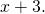Subtraction is often written as “less than,” “minus,” “decreased by,” “reduced by,” “subtracted from,” or “the difference of.” Subtraction statements are quite often written backwards. An example of this is “three less than an unknown number,” which is written as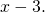Multiplication can be seen in written problems with the words “times,” “the product of,” or “multiplied by.”

Division is generally found by a statement such as “divided by,” “the quotient of,” or “per.”

Example 3.7.1

28 less than five times a certain number is 232. What is the number?

• 28 less means that it is subtracted from the unknown number (write this as −28)
• five times an unknown number is written as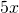• is 232 means it equals 232 (write this as = 232)

Putting these pieces together and solving gives: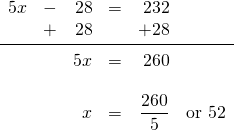Example 3.7.2

Fifteen more than three times a number is the same as nine less than six times the number. What is the number?

• Fifteen more than three times a number is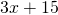or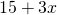• is means =
• nine less than six times the number is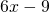Putting these parts together gives: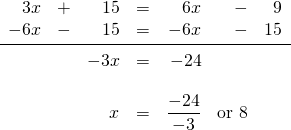Another type of number problem involves consecutive integers, consecutive odd integers, or consecutive even integers. Consecutive integers are numbers that come one after the other, such as 3, 4, 5, 6, 7. The equation that relates consecutive integers is: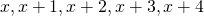Consecutive odd integers and consecutive even integers both share the same equation, since every second number must be skipped to remain either odd (such as 3, 5, 7, 9) or even (2, 4, 6, 8). The equation that is used to represent consecutive odd or even integers is: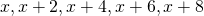Example 3.7.3

The sum of three consecutive integers is 93. What are the integers?

The relationships described in equation form are as follows: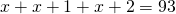Which reduces to: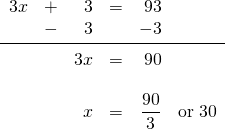This means that the three consecutive integers are 30, 31, and 32.

Example 3.7.4

The sum of three consecutive even integers is 246. What are the integers?

The relationships described in equation form are as follows: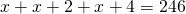Which reduces to: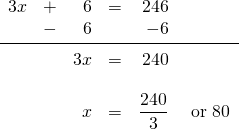This means that the three consecutive even integers are 80, 82, and 84.

# Questions

For questions 1 to 8, write the formula defining each relationship. Do not solve.

1. Five more than twice an unknown number is 25.
2. Twelve more than 4 times an unknown number is 36.
3. Three times an unknown number decreased by 8 is 22.
4. Six times an unknown number less 8 is 22.
5. When an unknown number is decreased by 8, the difference is half the unknown number.
6. When an unknown number is decreased by 4, the difference is half the unknown number.
7. The sum of three consecutive integers is 21.
8. The sum of the first two of three odd consecutive integers, less the third, is 5.

For questions 9 to 16, write and solve the equation describing each relationship.

1. When five is added to three times a certain number, the result is 17. What is the number?
2. If five is subtracted from three times a certain number, the result is 10. What is the number?
3. Sixty more than nine times a number is the same as two less than ten times the number. What is the number?
4. Eleven less than seven times a number is five more than six times the number. Find the number.
5. The sum of three consecutive integers is 108. What are the integers?
6. The sum of three consecutive integers is −126. What are the integers?
7. Find three consecutive integers such that the sum of the first, twice the second, and three times the third is −76.
8. Find three consecutive odd integers such that the sum of the first, two times the second, and three times the third is 70.